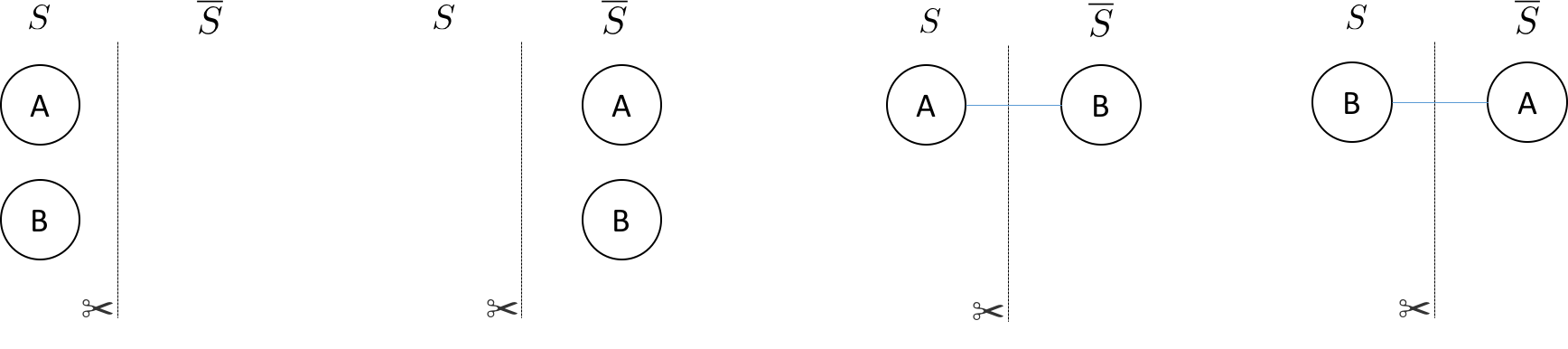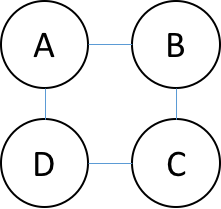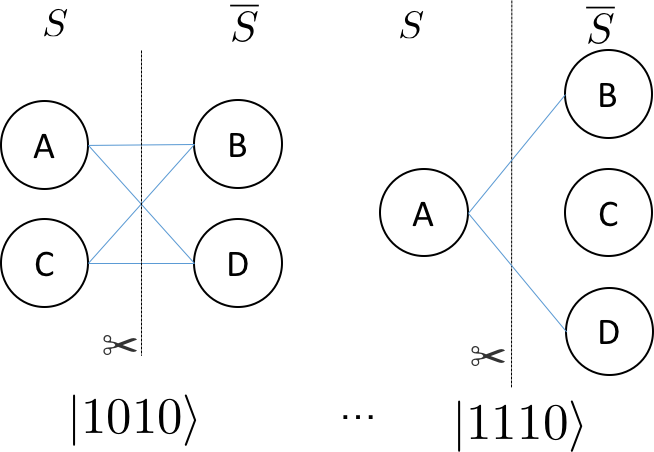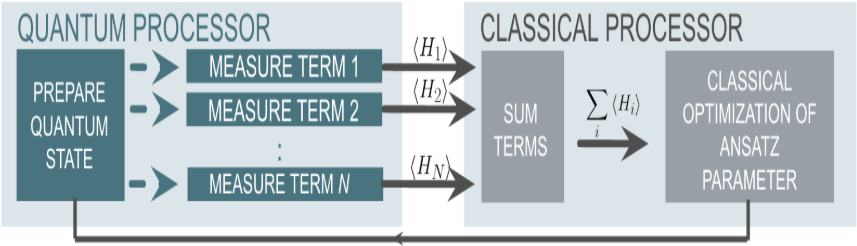6.1 QAOA算法¶

6.1.1 QAOA算法介绍¶

NP-Hard Problem解决方案¶

$\sum W_e\cdot Pr(e \in cut) = \frac{1}{2} \sum W_e$

$C_{ij} = 1/2(1-z_i z_j)$

$\sum_{ij}\frac{1}{2}(I-σ_i^z σ_j^z)$

$\begin{split}\widehat{H} = \frac{1}{2} (I-σ_z^1 ⊗σ_z^0 )= \begin{bmatrix}0& 0 & 0& 0\\ 0 & 1& 0 & 0\\ 0& 0& 1& 0\\ 0 & 0 & 0 & 0\end{bmatrix}\end{split}$

$(|00⟩, |01⟩, |10⟩, |11⟩)$

QAOA 通过从参考状态演变来识别 MAXCUT 哈密顿量的基态，该参考状态是哈密顿量的基态，它耦合总共 $$2^N$$ 个状态来构成成本哈密顿量的基底——成本函数的对角基底。对于 MAX-CUT，这是以 Z 为计算基底。

$\widehat{H}_τ= τ\widehat{H}_{ref}+ (1-τ)\widehat{H}_{MAXCUT}$

$\widehat{H}_{ref}= \sum_{i=0}^{N-1}\sigma ^{X}_{i}$

$|ψ_{ref}⟩=|+⟩_{N-1}⊗|+⟩_{N-2}⊗…⊗|+|+⟩_0$

H 0
H 1
...
H N-1

QPanda::QAOA要求用户输入参考哈密顿量和 MAXCUT 哈密顿量之间演化的近似步长。然后，该算法使用最大化成本函数的 quantum-variational-eigensolver 方法来变分地确定旋转的参数（表示为 β 和 γ ）。

$U = U(\widehat{H}_{\alpha1})U(\widehat{H }_{\alpha0})$

$U (\widehat{H}_{si})= U(\widehat{H}_{ref},β_i)U(\widehat{H}_{MAXCUT},γ_i )$

$U(\widehat{H}_{ref},β_i ) = e^{-iβ_{i}\widehat{H}_{ref}}$

$U(\widehat{H}_{MAXCUT},γ_i ) = e^{-iγ_{i}\widehat{H}_{MAXCUT}}$

$$U(\widehat{H}_{ref},β_i )$$$$U(\widehat{H}_{MAXCUT},γ_i )$$ 可以表示为一个短的量子线路。

$e^{−iβ_{i}\widehat{H}ref} = \prod_{n=0}^{1}e^{−iβ_{i}\sigma^{x}_{n}}$
H 0
RZ(beta_i) 0
H 0
H 1
RZ(beta_i) 1
H 1

$e^{-i\frac{γi}{2}(I-σ_1^Z⊕σ_0^Z)}$

X 0
U1(gamma{i}/2) 0
X 0
U1(gamma{i}/2) 0
CNOT 0 1
RZ(gamma{i}) 1
CNOT 0 1

$|β,γ⟩= e^{-iβ_1\widehat{H}_{ref}}\quad e^{-iγ_1\widehat{H}_{MAXCUT}}\quad e^{-iβ_0\widehat{H}_{ref}}\quad e^{-iγ_0\widehat{H}_{MAXCUT}}\quad |+⟩_{N-1,… ,0}$

$⟨β,γ|\widehat{H}_{MAXCUT} |β,γ⟩$

QPanda::QAOA利用经典量子混合方法，称为quantum-variational-eigensolver。量子处理器通常通过多项式操作来准备状态，然后使用该操作来评估成本。评估成本（ $$⟨β,γ|\widehat{H}_{MAXCUT}|β,γ⟩$$ ）需要进行许多准备和测量以生成足够的样本来准确地构建分布。然后，经典计算机再生成一组新的参数(β,γ)，以最大化成本函数。